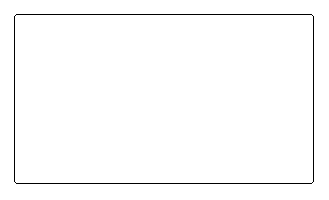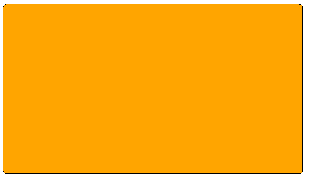# Rounded Rectangluar Regions

There are lots of examples that demonstrate how to draw a rectangle with rounded corners using GDI+ in .NET. Converting such a rectangle to a Region so that it can be filled or be used for the geometry of a window can have less than perfect results, though.

For example, here is one of the more concise methods for getting a rounded Rectangle:

```public static GraphicsPath GetRoundedRectangle(Rectangle rect, int rad)
{
int d = 2 * rad;
System.Drawing.Drawing2D.GraphicsPath gp =
new System.Drawing.Drawing2D.GraphicsPath();

gp.AddArc(rect.X, rect.Y, d, d, 180, 90);
gp.AddArc(rect.X + rect.Width - d, rect.Y, d, d, 270, 90);
gp.AddArc(rect.X + rect.Width - d, rect.Y + rect.Height - d, d, d, 0, 90);
gp.AddArc(rect.X, rect.Y + rect.Height - d, d, d, 90, 90);
gp.AddLine(rect.X, rect.Y + rect.Height - d, rect.X, rect.Y + d / 2);

return gp;
}
```

It's trivial to convert that GraphicsPath to a Region -- just call the appropriate Region constructor. You can then fill the region:

```var path = GetRoundedRectangle(rect, 3);
e.Graphics.FillRegion(Brushes.Orange, new Region(path));
```

If your corner radius is sufficiently large, you might not notice anything wrong with the result. With a value of 3, it is pretty obvious:The top left corner looks perfect:The bottom right corner is decidedly un-round:I started to wonder if all those round rectangle functions were wrong, but drawing the GraphicsPath itself shows that the code is correct:The problem is that when converting the GraphicsPath to a Region, it uses the inside of the GraphicsPath, so you lose the outside pixels on the sides, which you can see when drawing the path and then filling the region:It turns out to be a whole lot easier and effective to do a little p/invoke here:

```[DllImport("gdi32.dll")]
static extern IntPtr CreateRoundRectRgn(int x1, int y1, int x2, int y2, int cx, int cy);
// ...
var region = Region.FromHrgn(CreateRoundRectRgn(rect.X, rect.Y, rect.X + rect.Width, rect.Y + rect.Height, 3, 3));
e.Graphics.FillRegion(Brushes.Orange, region);
```

Here's the output from that code:It looks like a little larger corner radius is needed to get the same rounding, but otherwise it is perfect.